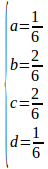# A simple method to classify CA // 一个简单的CA分类方法

Recall a CA ruleproject a circular-boundary state spaceback to it self, that iswhere, and more strictly, for simplicity we let, thusIn practical, we take n=20^2=400;

# 两种0/1序列的熵

1.考虑 s=[0,1]^d  如果d位里有a位为1,则考虑p=a/d  H(S)=p*log(p)+(1-p)log(p).

2.考虑同样的s, 令 x=a/d, p=p(x), H(S)=sum(p*log(p), for all s).

# 应用_用回归图对符号动力学进行半系统的分类_论可测变换或动力系统与度量函数的耦合暨微扰论的拓展(这里的单值用的都是协方差。)

# 记录_论可测变换或动力系统与度量函数的耦合_暨微扰论的拓展_1

2017/02/14 L，叁，F

L：抛出了对图论联通性和拓扑联通性之间的区别。L指出联通图可以自然导出一个最短路径（通过广度优先搜索），从而定义一个有离散拓扑的度量空间，但是图之联通不能导出最短路径对应的离散拓扑之联通。原因在于，由于这个拓扑是离散拓扑，所以这个拓扑对应的图显然不连通（只要图中的元素大于等于两个），而且由于道路/路径联通蕴含连通性，所以图也就不道路联通了。

L：总之，图论中最完全的信息，由边和顶点共同提供，可以人为地给图定义一些度量空间，但是这个度量在使用时要当心（叁：估计刘大师的意思是，图里的metric（比如由连接两点的最少的边的数量决定）并不是一个真正的metric space里的metric）。或者说是在套用metric space的结论时，要小心图论和拓扑空间之间术语的区别。

L：嗯其实是个metric，主要是连通性不一致可能会出错。譬如图论中的道路和拓扑空间就有点不一样。

F：让我重新表述一下我的问题：

# A partial derivation of the RK4 ODE-solver // RK4积分器的部分推导

RK4
is an ODE solver similar to euler’s method, with the iterator taking
the
form: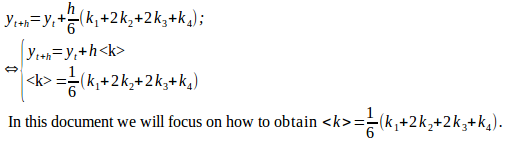RK-family
method relies on finding k_n iteratively, in RK4 the iteration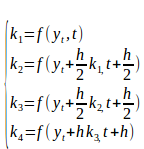However,
these relations
are
only useful

to derive k2, not from k1 directly, but
proxyed through
a complicated y. We now seek to eliminate this proxy. We wonder what
happened to the coefficients of k1 to k4. Thus, we should
taylor-expand k1 to k4, exploiting the aforementioned preserved
relation. Note this expansion has a 2D form if partial derivative is
taken. However we can exploit the principle of total derivative to
simplify the notation.(This is essentially dropping the dependency of
“f” on “y” to achieve a simpler notation).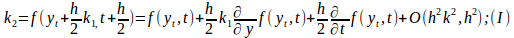By
the principle of total derivative: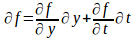Take
derivative wrt t: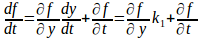Exchange
LHS with RHS, time both
sides
by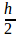gives: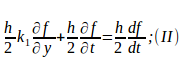substitue
( II ) into ( I ) gives: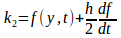Note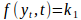,in
other words: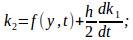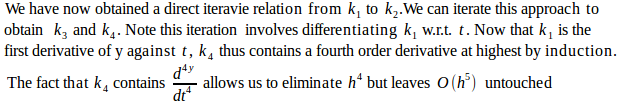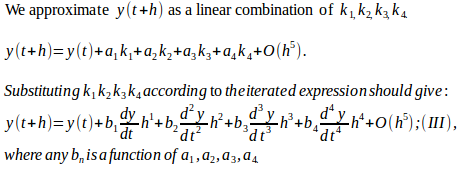Compare
(III) against well-known Taylor expansion of y( t+h ):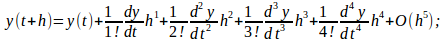We
obtain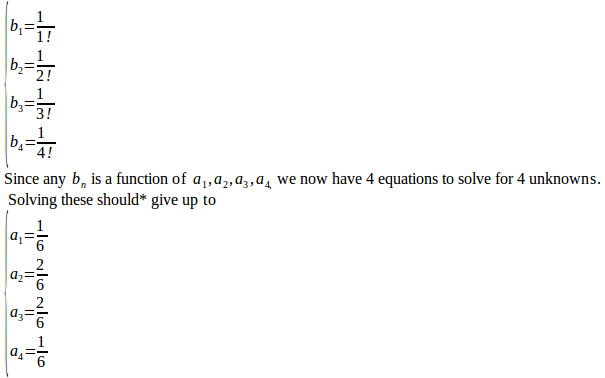*According
to Wikipedia, the full expansion of  (III) looks likeGiving
up to:and
thus